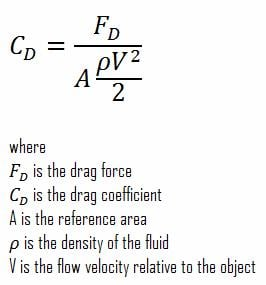# Calculating the Coefficient of Lift and Drag at various angles of attack in ANSYS

Hi,

I want to plot a graph of Coefficient of Drag for a 3D airfoil at various Angles of Attack.

I have done a simulation in ANSYS FLUENT with the Angle of Attack as the INPUT PARAMETER and the Drag force as the OUTPUT PARAMETER.

I should be using the formula shown below within the PARAMETER SET....MY QUESTION is this, what should I include as my reference area (S)? And how do you calculate it for various AoA?

Thank you.

• edited July 3

I understand the concept of the reference area from the link you shared. In this case, I am taking the projected area of the aerofoil looking at it directly from the leading edge.

Now, how do we calculate that (the projected area)? Can ANSYS automatically calculate it for various angles of attack?

Since the lift and drag forces can be Automatically generated within ANSYS, can the same be possible with their coefficients?

Thank you

• edited July 3

Yes it can: under Report-> Projected Areas

• edited July 5

Then how do we calculate the coefficients of lift and drag using this this area for various AoA

• edited July 6

By changing the reference area or you do it in third party or via UDF: if you have the force and you now the direction vector you want to look you can do everything outside of Fluent.

• edited July 14

Actually, I got the solution to my question: When creating the lift and drag coefficients, there is an option for setting them as output parameters. This would solve my problem. With the Cl and Cd set as output parameters, I can then auto-calculate them by only changing the AoA and the inlet velocity.

Regards.

• edited July 15

Perfect!